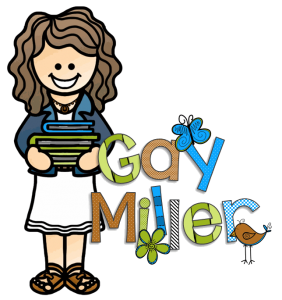# Math Resources ~ Math PropertiesLast summer I organized the math materials I had created in preparation for teaching a 5th-grade math class this school year. The result of this organization is several math blog posts.  You can find the updated math section of my website here. I also created four math products that I am selling on Teachers pay Teachers.

This post contains two free resources for teaching math properties. The first is a foldable organizer. The second is a Smart Notebook practice.## Math Properties Organizer

The organizer come in three versions:

• The first has lines where students write definitions. Students must also create samples for each math property.

• The second copy of the organizer contains the definitions with keywords missing for students to add. Students must also create samples for each property.

• The third copy is completed. It may be used as an answer key. The organizer may be displayed on a SmartBoard for students to copy. A great use for the completed organizer is for differentiated instruction. The completed organizer may also be used for students who were absent.### Common Core State Standards

CCSS.Math.Content.3.OA.B.5 Understand properties of multiplication and the relationship between multiplication and division.

CCSS.Math.Content.6.NS.B.4 Use the distributive property to express a sum of two whole numbers 1-100 with a common factor as a multiple of a sum of two whole numbers with no common factor. For example, express 36 + 8 as 4 (9 + 2).

### The Properties

#### Commutative Property

You can swap numbers around and still get the same answer when you add or multiply.

[interchangeable in order]

Examples

4 x 2 = 2 x 4

#### Associative Property

In addition and multiplication, you can group a series of numbers in any order and get the same answer.

[grouping doesn’t matter]

Examples

(2 x 3) x 4 = 2 x (3 x 4)

#### Distributive Property

A characteristic of numbers that allows you to multiply a group of numbers and get the same answer you would get if you multiplied each member of the group and then combined the answers.

4 x (3 + 5)

(4 x 3) + (4 x 5)

#### Identity Property

Numbers that keep their expressions the same.

Multiplicative/Division Identity Property:

You can multiply one [or divide by one] to any number and your number will stay the same.

Example

5 x 1 = 5

You can add zero [or subtract zero] to any number and your number will stay the same.

Example

5 -0 = 0

## Here are the math products I am selling on Teachers pay Teachers: